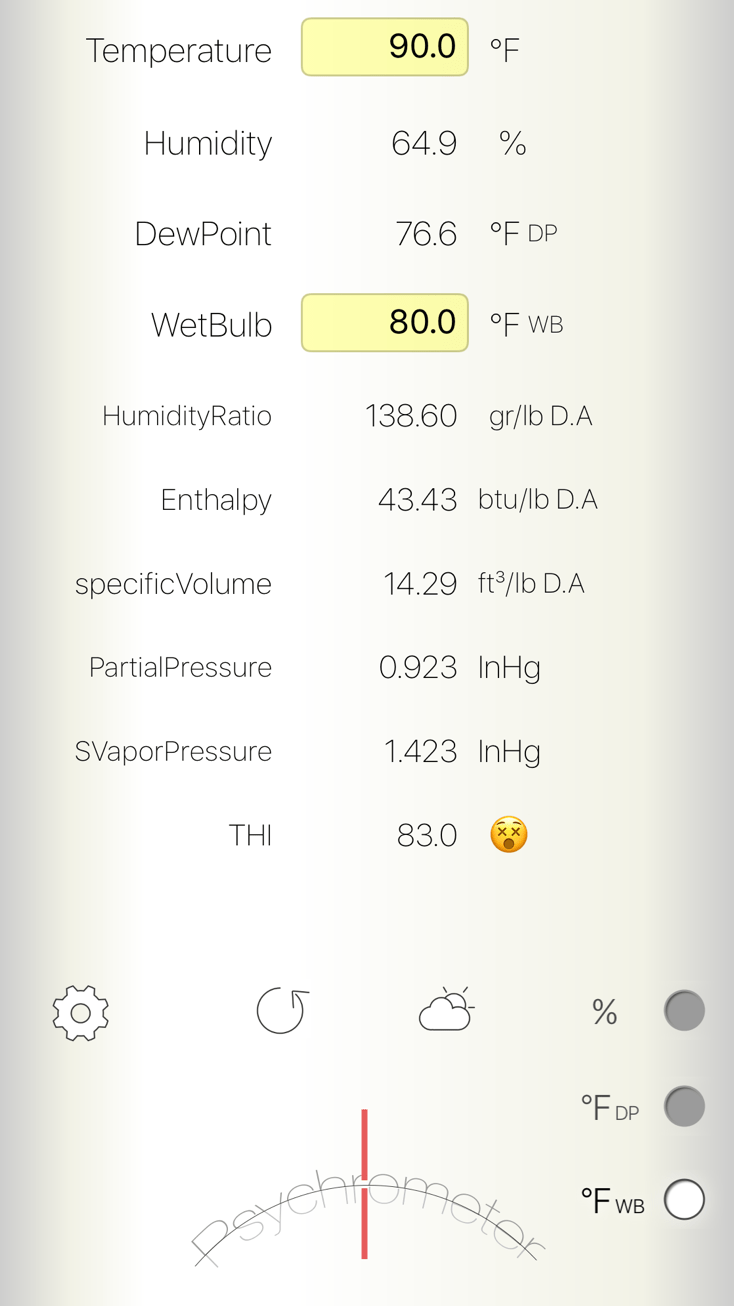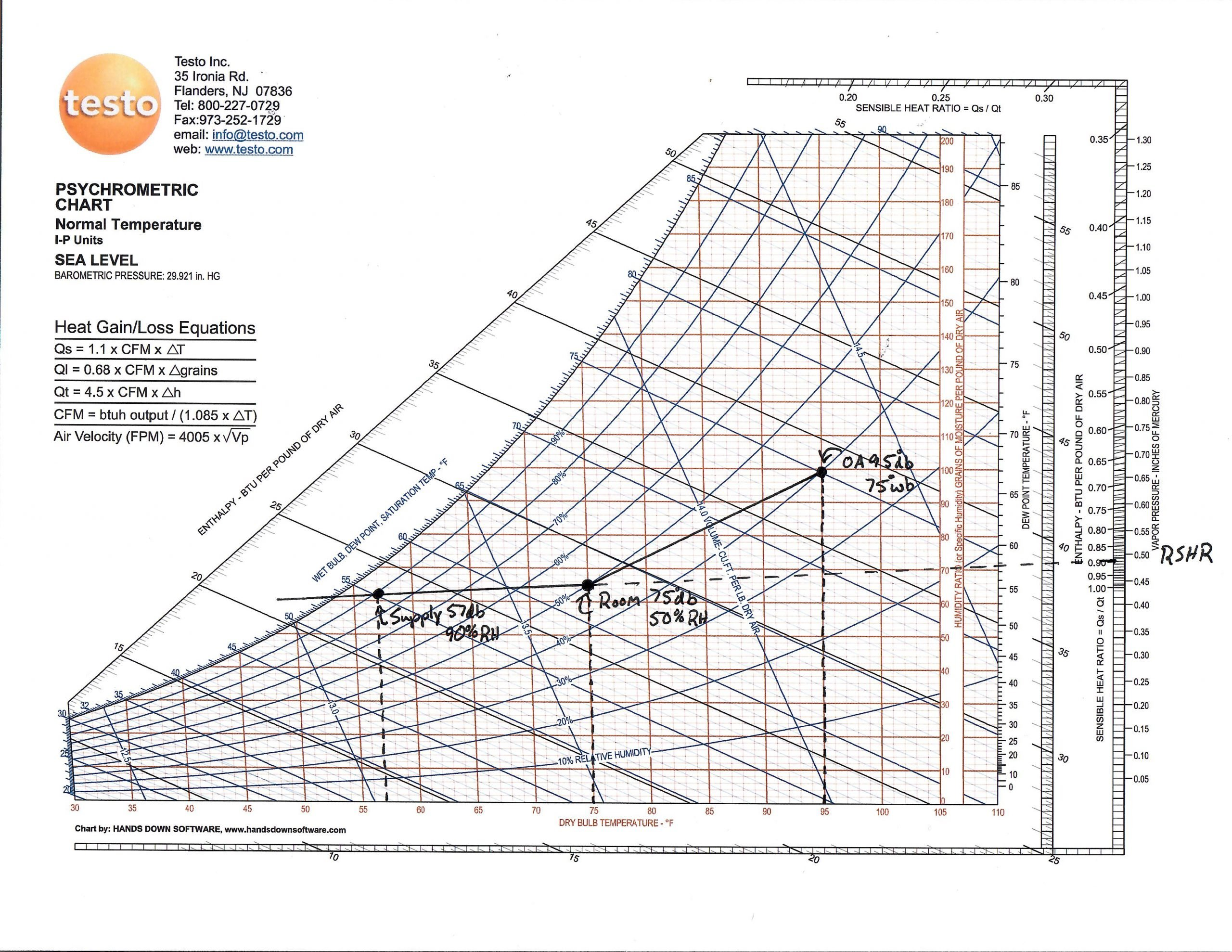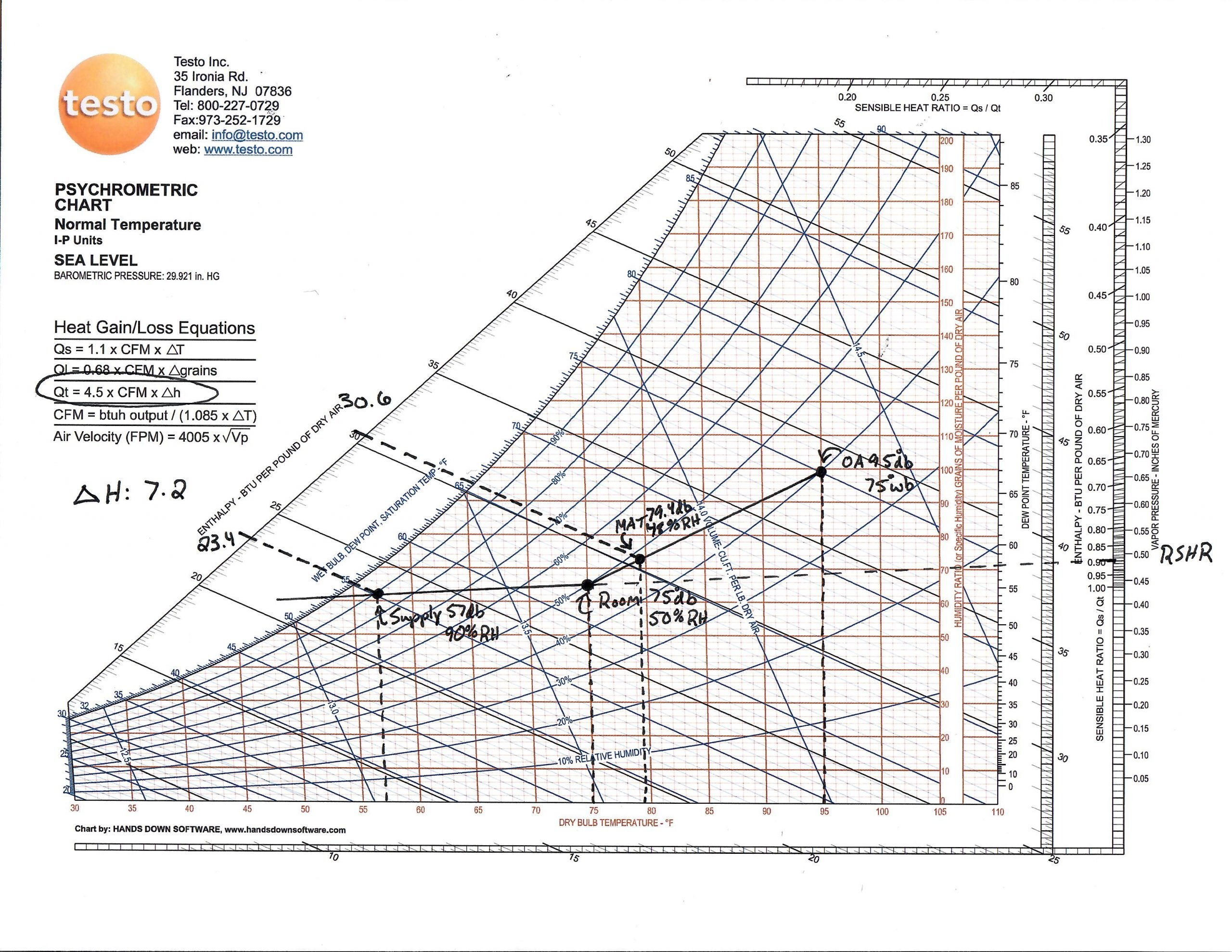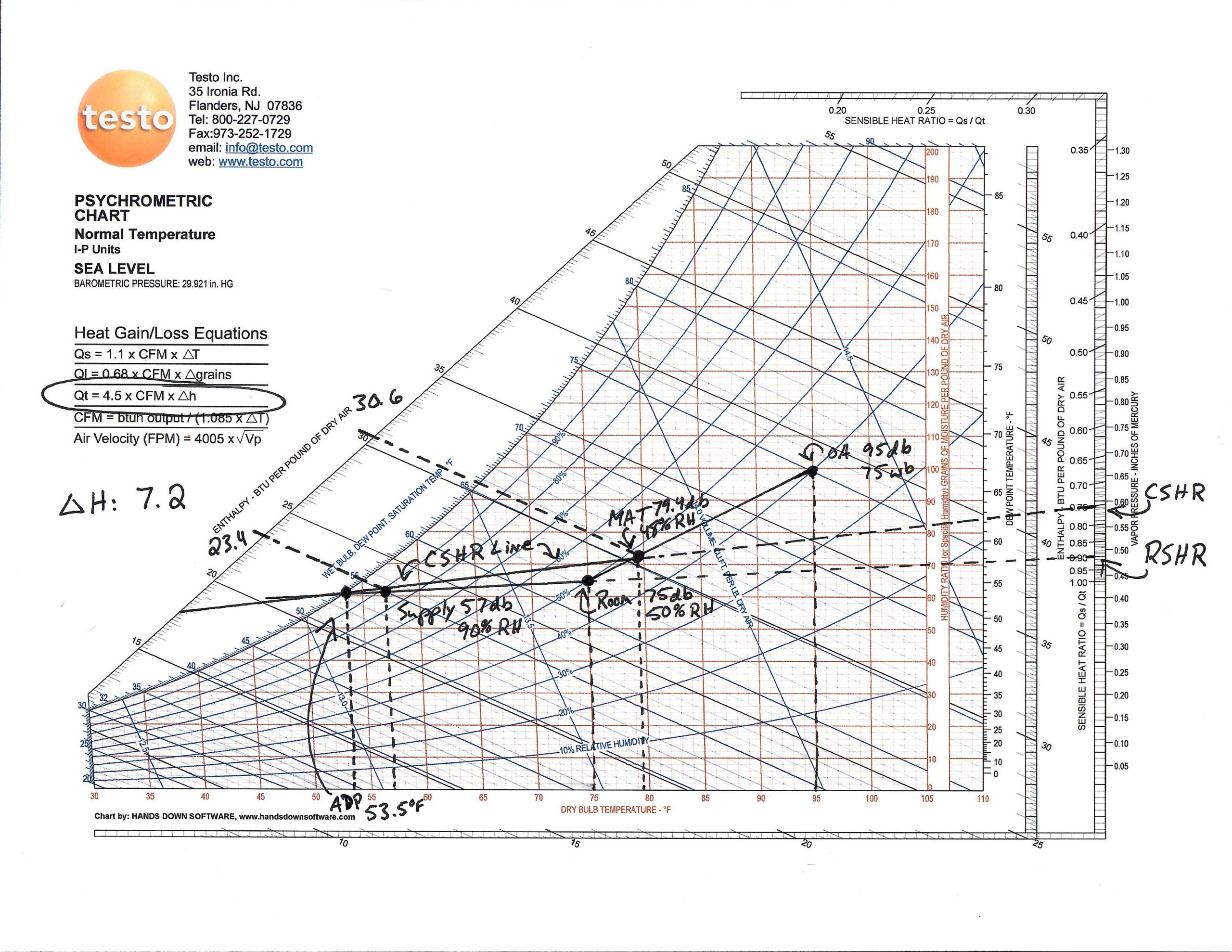## Coil SHR, Ventilation, and Bypass Factor – Advanced Psychrometrics Part 2This is the second article in a three-part series, where Advanced Psychrometrics are explored. The source material for each of the articles in this series is ACCA Manual P Sections 3, 4, and 5. This article is based on information found in Section 4.

If you followed the previous Advanced Psychrometrics article, you now know how to use a Psych Chart to plot a Room Sensible Heat Ratio (RSHR) Line, and how to calculate Design Room CFM. However, if you followed that exercise, you will note the absence of real-world variables, such as ventilation and bypass factors. Equipment Sensible Heat Ratios are almost never an exact match to the RSHR. This exercise will account for these variables, and walk you through how to plot these properties on a Psychrometric Chart.

It is worth reminding you that this is an exercise to help illustrate the complexities of psychrometry in the real world. This may not always be a practical method utilized in the design process.

When outdoor ventilation air is mixed with return air before the equipment coil, the equipment is exposed to latent and sensible loads beyond that of just the conditioned space. This characteristic causes the Coil Sensible Heat Ratio (CSHR) to alter from the RSHR. Remember, the Room Design Conditions will be met only when the supply air properties fall on the RSHR Line. With two different SHRs, we no longer have the luxury of choosing any supply condition we wish. The supply air must be able to cool and dehumidify the space. It also must now compensate for the additional load introduced by the ventilation air. Therefore, the only supply condition that will satisfy the Room Design Condition is the point at which both the RSHR Line and CSHR Line meet on the Psych Chart.

To plot the RSHR Line should be a breeze at this point. For a review on that process, and the first part of this article series, CLICK HERE.

The construction of the CSHR Line, however, is a bit more involved. There is a little trial and error in the construction of the CSHR Line. It’s not impossible, of course, and with practice, you get pretty good at nailing it on the first try. Here’s why a trial and error process is required in order to plot the CSHR Line:

• The location of the CSHR Line is determined by the Mixed Air Condition (MAT) and CSHR
• The CSHR and the MAT can’t be plotted without knowing the percentage of Outdoor Air (OA)
• The percentage of OA can be calculated only when the supply CFM is known.
• The supply CFM can be calculated only when the ΔT between the room return and supply is known, which is determined by the intersection of the CSHR and RSHR Lines
• The CSHR Line is the line we are solving for; therefore, it is unavailable.

This is why a trial and error process is required. Simply put, we’re going to use an estimated guess as to what we think our supply air condition will be, then follow the process until we can determine if our selection actually results in the intersection of the CSHR and RSHR Lines. To help aid in the accuracy of your guess, keep in mind that, on average, a Direct Exchange Fan Coil can provide supply air temperatures which may fall between 14-25 degrees below the space temperature at typical relative humidities between 80% and 95%.

To begin this exercise, let’s start with some basic information, which will ALWAYS be available to you from a quality load calculation. This information can be plotted on the Psych Chart with complete certainty:

Room Sensible Heat: 21,700 BTUh

Room Latent Heat: 2,300 BTUh

Room Total Heat: 24,000 BTUh

RSHR: 0.90

Room Design Condition: 75℉ db / 50% RH

Outdoor Design Condition: 95℉ db / 75℉ wb

Ventilation Required: 245 CFM

In this scenario, a Room-to-Room load calculation has been done on a home. The RSHRs have all been averaged together for a mean room sensible heat ratio. We can go ahead and plot what we can on the chart:Let’s select a 57℉ supply temperature at about 90% RH. Now we can determine the Supply CFM. Since the RSHR is the average of the entire home, the Supply CFM will equal the total system CFM.

CFM = Room Sensible Load ÷ (1.08 x ΔT)

21,700 ÷ (1.08 x 18) = 1,116 CFM

Now that we know the Supply CFM, we can calculate the percentage of ventilation air.

Ventilation =  245 CFM ÷ 1,116 CFM

Ventilation = 22%

We have a good bit of information here now, but the math starts to get a little confusing without explanation. We now know that 22% of Outdoor Air (at 95℉ db / 75℉ wb) will be mixing with the remaining 78% Return Air (at 75℉ db / 50% RH). To calculate the Mixed Air Condition, complete the following equation:

MAT = (0.22 x 95℉) + (0.78 x 75℉)

MAT = 20.9℉ + 58.5℉

MAT = 79.4℉

We can now plot the Mixed Air Condition on the Psych Chart.At this point, we have everything we need to construct the Coil Sensible Heat Ratio Line. If you notice on the Psych Chart, there is a list of helpful formulas to the left of the page. We need to solve for Total Coil Heat Load (Qt) if we are to determine Coil Sensible Heat Load (Qs) and CSHR. To do that, we need to figure out the change in enthalpy (ΔH). Enthalpy is heat energy in BTUs per pound of dry air.

ΔH = 30.6 – 23.4

ΔH = 7.2

Now let’s plug our ΔH into the Total Coil Heat Load calculation. (4.5 here is Air Density x Run Time in minutes. 0.075 x 60 = 4.5)

Qt = 4.5 x CFM x ΔH

Qt = 4.5 x 1,116 x 7.2

Qt = 36,158 BTUh

Solve for Coil Sensible Heat Load. To do this, make sure you are using the entering air condition the equipment will actually see: MAT.

Qs = 1.08 x CFM x ΔT

Qs = 1.08 x 1,116 x 22.5

Qs = 27,119 BTUh

We can finally solve for Coil Sensible Heat Ratio at this point:

CSHR = 27,119 BTUh ÷ 36,158 BTUh

CSHR = 0.75

We can now plot the CSHR Line on the Psych Chart.If you look closely, you may be thinking, “Wait a second, the CSHR Line does not intersect with the RSHR Line.” You would be absolutely correct. This is why the trial and error solution is necessary. However, if you notice, the CSHR Line is extremely close to our selected supply temperature. The CSHR Line is just slightly above the RSHR Line.

What does this mean?

We can still use the design CFM and supply condition, and the equipment will satisfy the sensible load, but will maintain a slightly higher humidity level in the space than what was designed. Take a look at the actual grains of moisture for the Mixed Air Condition in comparison to the Supply Air off the coil at 57℉.The equipment will be able to dehumidify from 73 grains of moisture/lb of dry air down to 63 grains of moisture, rather than the ideal 62 grains. We’re talking about a difference of 1 grain of moisture. This can be acceptable, and the difference likely unnoticeable. In cases where a coil selection will not match the latent load requirements of a space, a viable option would be to add supplemental dehumidification to deal with the remaining latent load. Ultra-Aire Ventilating Dehumidifiers are an excellent option, and will also help lessen the additional latent load from the ventilation air.

Lastly, let’s talk about Bypass Factor. Remember, the ideal supply temperature would be the apparatus (equipment) dew point. However, there is a small percentage of air that will bypass the coil and not transfer its heat to the coil. This can be calculated using the known apparatus dew point. The Bypass Factor formula is as follows:

Bypass Factor = (Supply Air Temperature – Apparatus Dew Point) ÷ (Mixed Air Temperature – Apparatus Dew Point)

Bypass Factor = (57 – 53.5) ÷  (79.4 – 53.5)

Bypass Factor = 3.5 ÷ 25.9

Bypass Factor = 0.14

At this point, you would need to look up a manufacturer’s extended performance data for their equipment to ensure that the coil you select will meet a sensible capacity of 27,119 BTUh and a total capacity of 36,158 BTUh at 1,116 CFM, with an entering condition of 79.4℉ db / 65.6℉ wb and an outdoor condition of 95℉ db / 75℉ wb. Let me translate that to something you might actually see on a Performance Table:

Entering Air Condition: 80℉ db / 67℉ wb

Outdoor Air Conditions: 95℉ / 75℉ wb

Total Capacity: 36,000 BTUh

Sensible Capacity: 27,000 BTUh

Airflow: 1,100 CFM

If you can select a coil that will match these criteria, you will be able to maintain an indoor air condition that is nominally close to your design.

To see how this chart would look in another scenario (without going through the step-by-step process), here is a psych chart based on my house and ASHRAE Design Conditions:

Room Sensible Heat: 16,800 BTUh

Room Latent Heat: 7,200 BTUh

Room Total Heat: 24,000 BTUh

RSHR: 0.70

Room Design Conditions: 75℉ db / 50% RH

Outdoor Design Conditions: 90℉ db / 80℉ wb

Ventilation Requirement: 46 CFMIn this case, my selected supply air condition happened to fall perfectly at the intersection of the CSHR and RSHR Lines. The tricky part, however, is finding a coil that will meet the sensible and latent heat requirements under the design conditions. I would need to look for a coil with 18,000 BTUh sensible capacity and 29,000 BTUh total capacity. I’d have to settle for a 2.5 ton (30,000 BTUh) coil with a close CSHR under design conditions, and potentially add supplemental dehumidification. (A Carrier FB4C–030 would actually fit the bill quite nicely.) Remember, the equipment selection performance table will have actual capacities that differ from the nominal rating; thus, care must be taken when using manufacturer performance tables to select equipment.

If you’ve made it to the end of this exercise, congratulations: you are as nerdy as they come! I hope this helps illustrate the complexities of psychrometrics. If nothing else, the take away should be a new-found respect for psychrometrics, and its integration into a technician’s daily diagnostic toolbag.

Stay tuned for Part 3, where we will dive into ACCA Manual P, Section 5. There we will learn how to account for duct gains, and how reheat dehumidification looks on a Psych Chart.

–Kaleb Saleeby

This site uses Akismet to reduce spam. Learn how your comment data is processed.

Scroll to top
Translate »

### Daily Tech Tip

Get the (near) daily Tech Tip email right in your inbox!